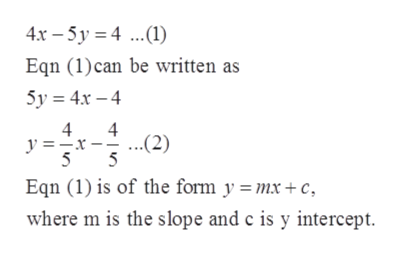# find an equation of the line that is perpendicular to the line 4x - 5y = 4 and passes through the point (7, -3)

Question
3 views

find an equation of the line that is perpendicular to the line 4x - 5y = 4 and passes through the point (7, -3)

check_circle

Step 1

Given:

The given equation of line is 4x-5y=4 …. (1)

Step 2

To find the equation of line which is perpendicular to 4x-5y=4 and passes through the point (7, -3), first find the slope of line 4x-5y=4.

Reduce the equation (1) into slope-intercept form.help_outlineImage Transcriptionclose4x-5y 4. Eqn (1) can be written as 5y 4x-4 4 4 ..(2) 5 y =x 5 Eqn (1) is of the form y =mx+ c where m is the slope and c is y intercept fullscreen
Step 3

On comparing Equation (1) and (2) m=4/5 and c=-4/5.

Therefore, slope of equation (1) is 4/5.

Since slope of perpendicular lines are negat...

### Want to see the full answer?

See Solution

#### Want to see this answer and more?

Solutions are written by subject experts who are available 24/7. Questions are typically answered within 1 hour.*

See Solution
*Response times may vary by subject and question.
Tagged in

### Equations and In-equations